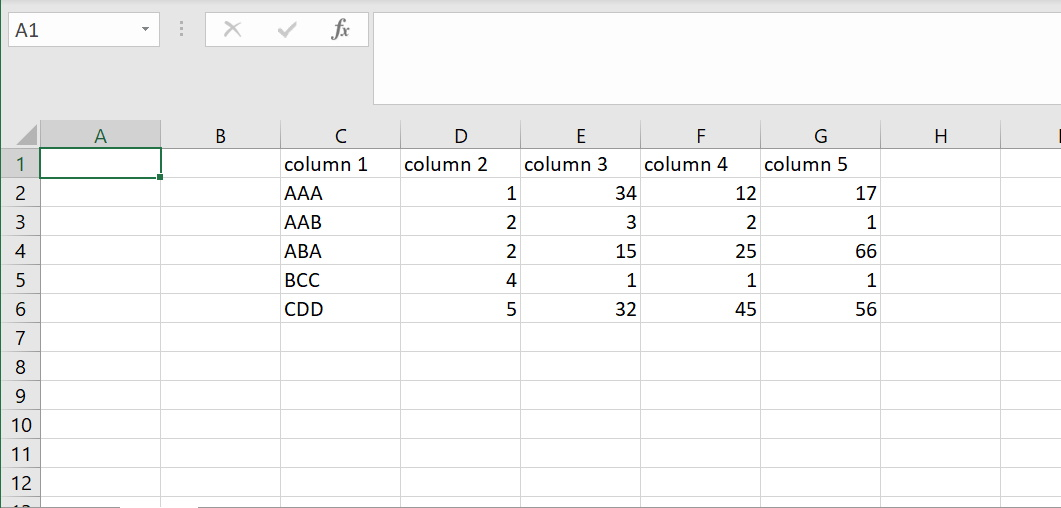# Read xlsx files in R

The most common way to get data from an Excel spreadsheet and import it into R is to use Excel to save the data into a more conventional format, like `.csv`. But if you need to import `xlsx` files repeatedly, a quicker option, like using a package to import Excel files directly into R, is preferred.

Many packages in R have the capabilities to read `xls`/`xlsx` files. One of them is `readxl`. This package has fewer external dependencies than other packages, making it easy to install in almost any operating system. The most straightforward way to add it to your R environment is to install the whole `tidyverse` library with the following command:

``````install.packages("tidyverse")
``````

But since it is not a core `tidyverse` library, it still needs to be loaded explicitly with the `library(readxl)` command. Then you will be able to import an Excel file with the `read_excel` function, like this:

``````MySheet <- read_excel("ExcelFile.xlsx")
``````

In the previous example, we import the contents of a spreadsheet called `ExcelFile.xlsx` into a data frame called `MySheet`.

## Let the user specify the file to import

If you don’t know the Excel file location in advance and you want to let the user pick the file to read, you can use `file.choose()` instead of explicitly specifying the file path:

``````MySheet <- read_excel(file.choose())
``````

The `read_excel()` function has other parameters to specify a particular sheet to import or specify a value representing `NAs` instead of blank cells. In this example, we are importing a sheet called `data` and indicating that cells with the content `NA` will be interpreted as `NAs`:

``````MySheet <- read_excel("ExcelFile.xlsx", sheet = "data", na = "NA")
``````

## Use the `openxlsx` library to read xlsx file in R

Another package you can use to read Excel files into R is `openxlsx`. This package simplifies reading and writing xlsx files and, like `readxl`, and doesn’t depend on Java, Perl, or other external libraries. Using `Rcpp`, `openxlsx` provides fast read/write times even for huge files. This library works fine with xlsx files, but it doesn’t support older xls files.

`Openxlsx` provides the `read.xlsx` function that creates a data frame with the data read from the imported file. It has many options to specify if the area to import contains row and column names, the name or number of the worksheet to import, the rows and columns to import, among many others.

In the following example, we install the package `openxlsx` and opening the library. Then, we use the options in `read.xlsx` to import rows 2, 4, and 6 and columns 5 through 7 from sheet 1 of a file named Data.xlsx with this data:After that, we print the contents of the resulting data frame to the console. Since we don’t read the column names from the file, we set the parameter `colNames` to `FALSE`. R will automatically assign a default sequence of names: `X1`, `X2`, `X3` to the columns in the imported data frame. Note that we can specify which rows and columns to import by specifying them with vectors:

``````install.packages("openxlsx")
library("openxlsx")
MyData <- read.xlsx(xlsxFile="c:/Tmp/Data.xlsx", sheet = 1, rows = c(2, 4, 6), cols = 5:7, colNames = FALSE)
MyData
``````

Output:

``````  X1 X2 X3
1 34 12 17
2 15 25 66
3 32 45 56
``````

For further reading, check the official openxlsx documentation.